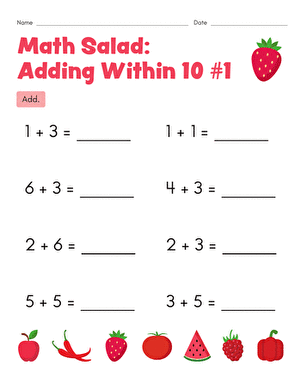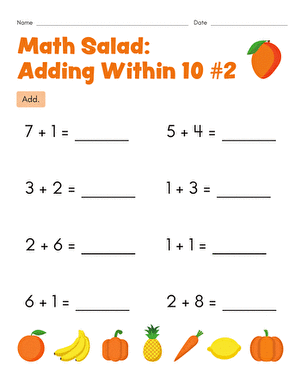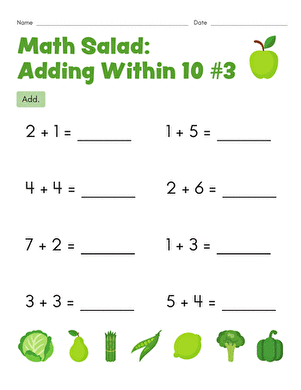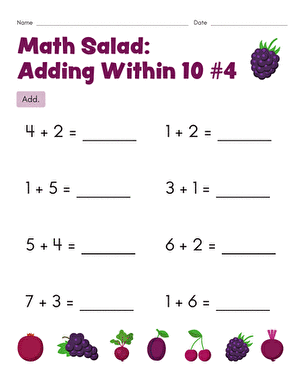As your first grader digs into some "maths salad," he will fine tune those important addition skills and strengthen his ability to perform basic mental maths.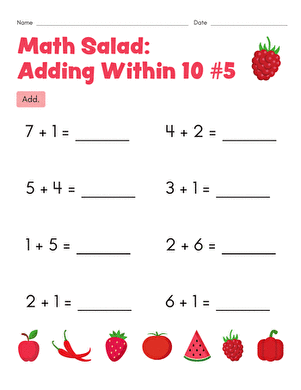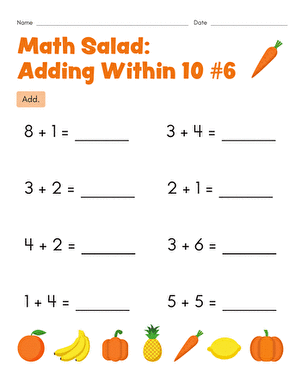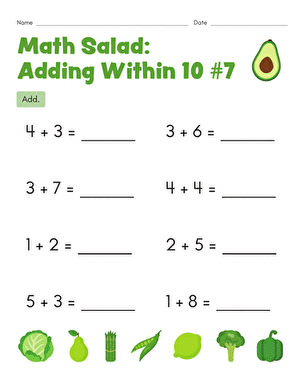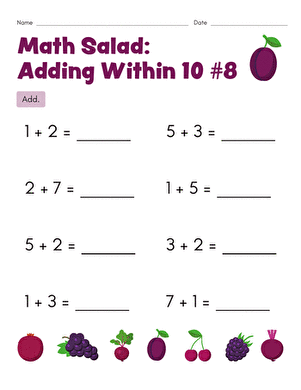This charming maths salad worksheet is so colorful and fun that your first grader will actually want to learn maths facts!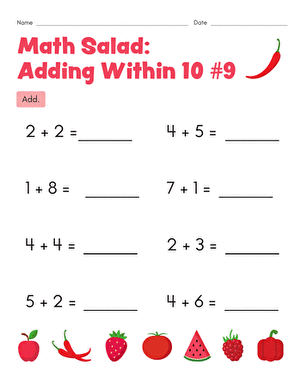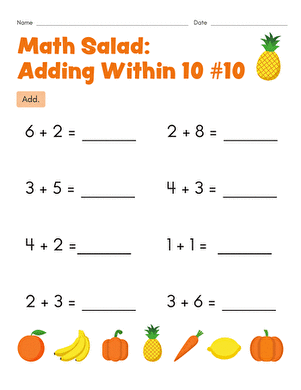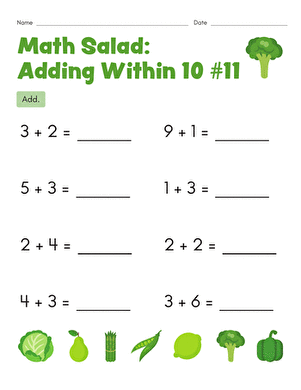Make solving addition problems fun with this fruit-filled year two maths worksheet! Your child will solve addition problems that add up to 10 or less.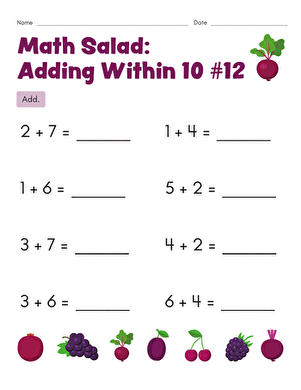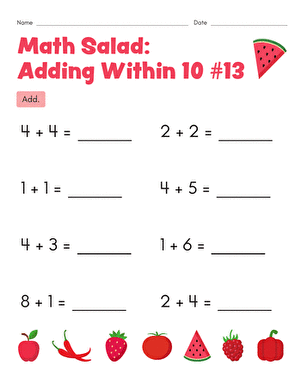Introduce your child to beginning arithmetic with this year two maths worksheet. To complete it, your child will solve single-digit addition problems.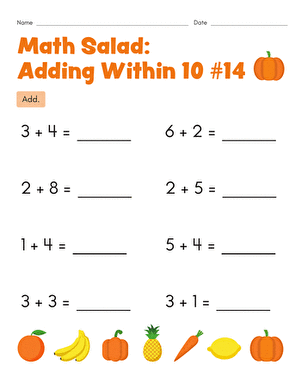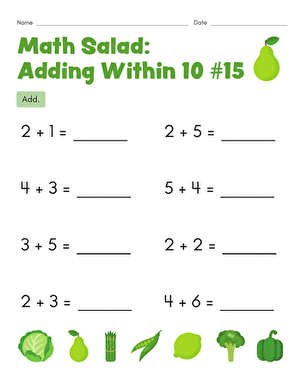Tap into the mathematical potential of fruit salad with this year two addition worksheet.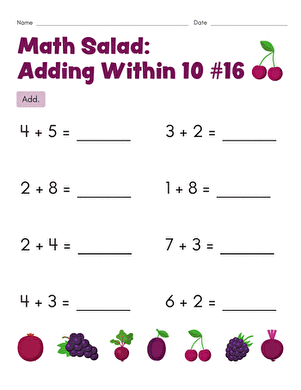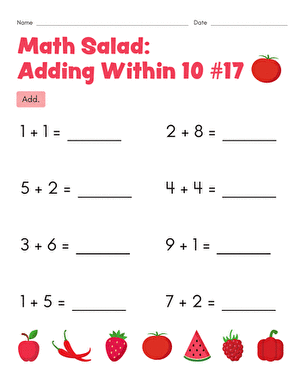Your child will get a head start on maths with this year two maths worksheet covering single-digit addition problems that add up to 9 or less.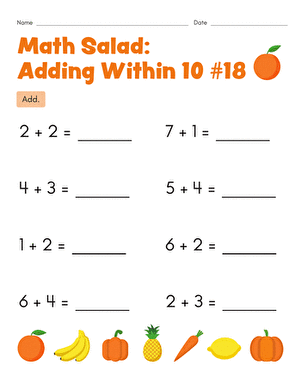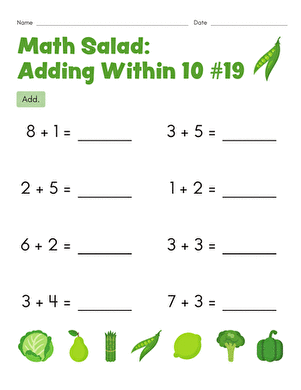Explore the educational side of fruit salad with this fun and fruity maths worksheet covering single-digit addition problems that add up to 9 or less.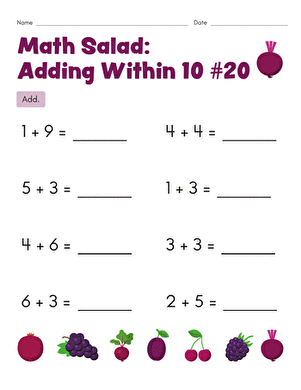Covering single-digit addition problems that add up to 9 or less, this year two maths worksheet offers a good introduction to beginning arithmetic.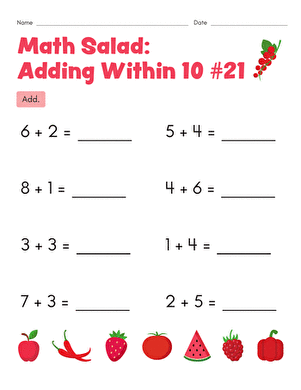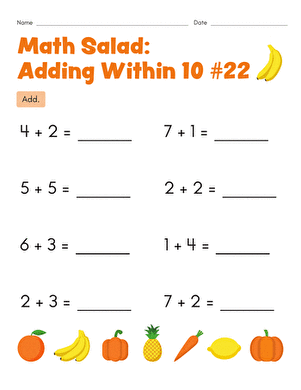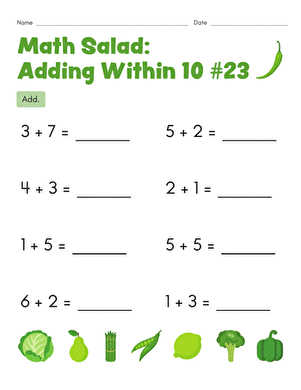As your first grader chomps away at these fruit-themed addition equations, he will strengthen his understanding of addition and his mental maths ability.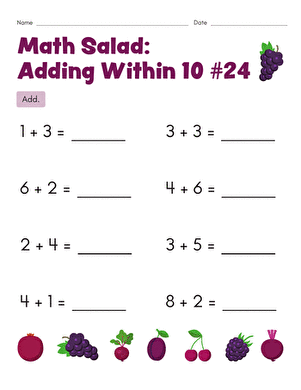Give your first grader an extra helping of "maths salad," and watch her improve her mental maths ability while fine tuning her understanding of basic addition.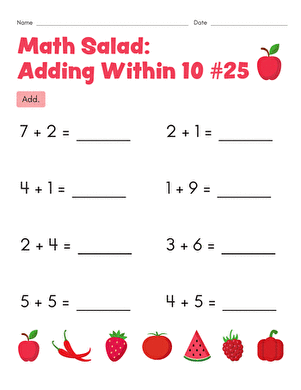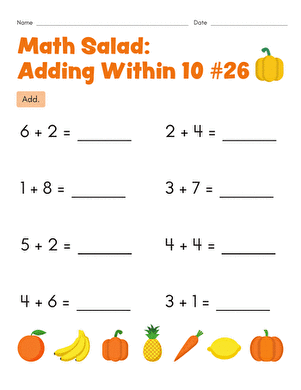This fruit-filled year two maths worksheet offers a healthy dose of mental nutrition. To complete it, your child will solve single-digit addition problems.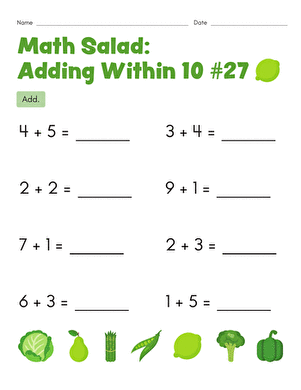Introduce your child to beginning arithmetic with this fun and fruity year two maths worksheet covering single-digit addition problems with sums up to 9.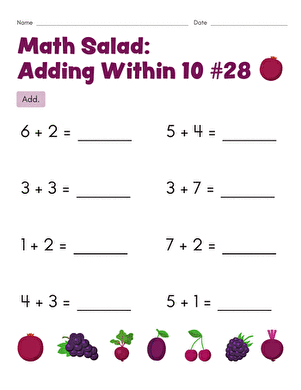Covering single-digit addition problems, this year two maths worksheet offers an approachable introduction to maths beyond counting.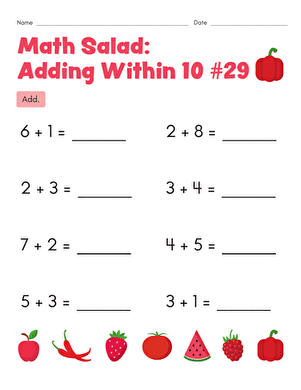In this fruity year two maths worksheet, your child will solve single-digit addition problems with sums up to 9.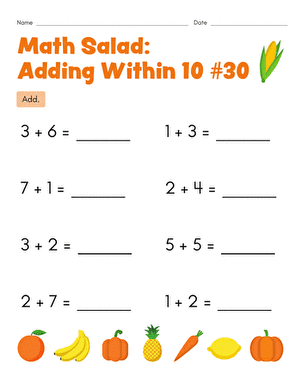In this fruit-themed year two maths worksheet, your child will solve single-digit addition problems with sums of 9 or less.

Create new collection

0

### New Collection>

0Items

What could we do to improve Education.com?